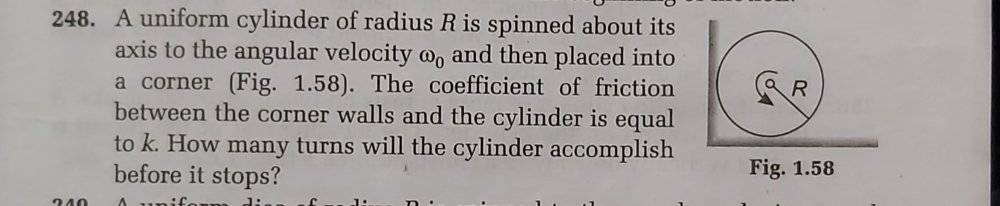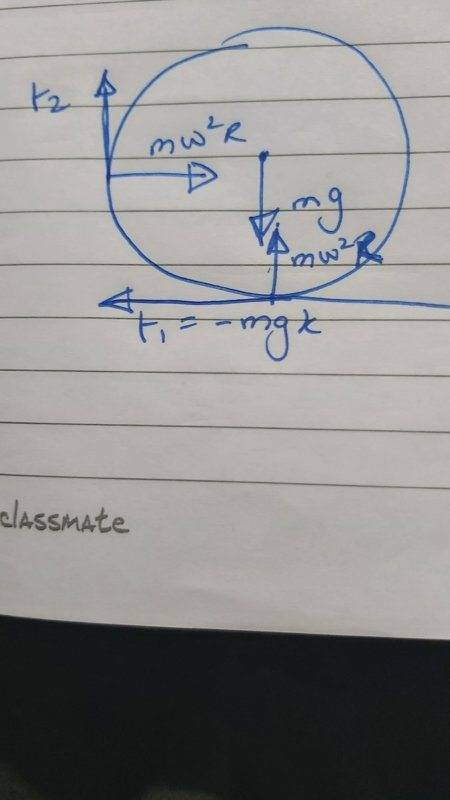# Question of Rotational motion

Pi-is-3
Homework Statement:
Find the number of revolutions by the cylinder.
Relevant Equations:
$$\tau = I \alpha$$
Change in Kinetic energy = Work done
Hello, I'm stuck in this rotational motion problem (advanced high school level).Source: Problems in General Physics- IE Irodov

My attempt(s):

First I tried using work done by the moment of friction (mgkR) and equated it with change in KE.

I got the answer as ## \frac{R (\omega_0)^2}{8 \pi g k} ##.

However, the correct answer is ## \frac{(1+k^2)R (\omega_0)^2}{8 \pi g k(1+k)} ## .

The first mistake I thought of is not including centripetal, but the centripetal is varying with ## \omega ## and whenever I solve it that way I am not even getting close to the answer. Using ## \tau=I \alpha ## is getting nowhere either.

Any hint is appreciated. Thank you.

Homework Helper
Gold Member
2022 Award
Homework Statement:: Find the number of revolutions by the cylinder.
Relevant Equations:: $$\tau = I \alpha$$
Change in Kinetic energy = Work done

Hello, I'm stuck in this rotational motion problem (advanced high school level).

View attachment 256836
Source: Problems in General Physics- IE Irodov

My attempt(s):

First I tried using work done by the moment of friction (mgkR) and equated it with change in KE.

I got the answer as ## \frac{R (\omega_0)^2}{8 \pi g k} ##.

However, the correct answer is ## \frac{(1+k^2)R (\omega_0)^2}{8 \pi g k(1+k)} ## .

The first mistake I thought of is not including centripetal, but the centripetal is varying with ## \omega ## and whenever I solve it that way I am not even getting close to the answer. Using ## \tau=I \alpha ## is getting nowhere either.

Any hint is appreciated. Thank you.
Did you draw a force diagram? If so please post that as an image, or list the forces involved.
Remember, both surfaces have friction.

•etotheipi
You said you tried using work; can you devise an expression relating the number of revolutions to the angular work done by the two frictional forces?

Homework Helper
Gold Member
2022 Award
You said you tried using work; can you devise an expression relating the number of revolutions to the angular work done by the two frictional forces?
I think @Pi-is-3's error is in determining those forces.

•Pi-is-3 and etotheipi
Pi-is-3
I am unable to find the frictional force on the left wall.

I am unable to find the frictional force on the left wall.

If you draw a free body diagram of the situation, what equations can you write down involving the normal forces, frictional forces and weight?

•Pi-is-3 and CPW
CPW
In determining the reaction force on the cylinder by the wall, consider that there is no translational acceleration of the center of mass of the cylinder.

•Pi-is-3
Pi-is-3Ignore the minus sign behind mgk. Their has to be some involvement of centripetal force in friction, but I am not sure how, because it is directed towards the center, and not towards the ground. I know I have probably done it very wrong. I'm sorry if it's too bad.

Pi-is-3
Oh wait, maybe the normal reaction at ground isn't mg. I have had an idea, let me try it out first.

•etotheipi
Pi-is-3
In determining the reaction force on the cylinder by the wall, consider that there is no translational acceleration of the center of mass of the cylinder.
Got it! Since their is no translational motion, the normal reaction from ground+ frictional on the left wall= mg.

And, friction on ground = Normal reaction from the left wall.

Done!

Pi-is-3
Thank you everyone, this problem has been solved.